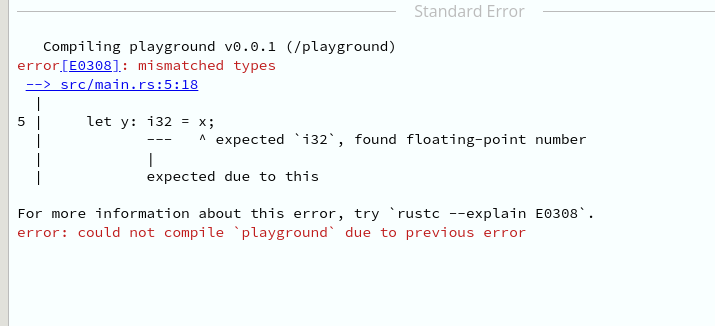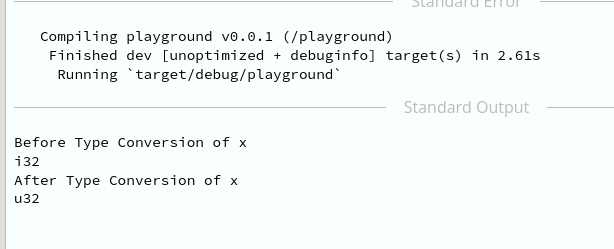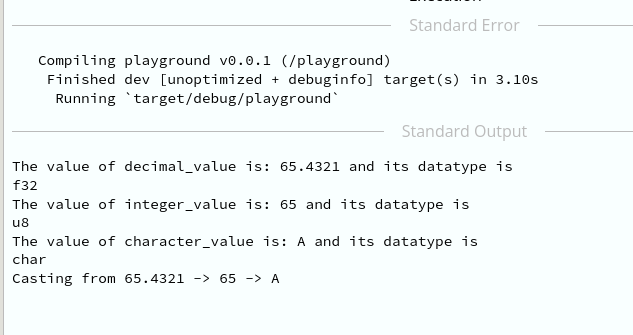# Rust – Casting

• Last Updated : 07 Mar, 2022

Type Conversion or casting in Rust is a way to convert one type to another. As its name suggests, type conversion is the process of converting variables from one data type to another. So that, the compiler treats the variable as a new datatype. Rust doesn’t allow us to implicitly convert the datatype between primitive types.

The primitive types are i8, i16, i32, i64, u8, u16, u32, u64, f32, f64, char, and bool. Here i8, i16, i32, i64 are signed integer types and u8, u16, u32, u64 are unsigned integer types. f32 and f64 are floating-point types. char is a single character type and bool is a boolean type.

The term implicitly means that the compiler does not require us to write the code to convert the data type. The compiler does this automatically. But rust doesn’t allow us to implicitly convert the datatype between primitive types. We have to explicitly convert the data type using the as keyword.

### Type of casting:

1. Implicit type casting
2. Explicit type casting

### 1. Implicit Type Casting:

Implicit typecasting is the process of converting one data type to another. The compiler does this automatically.

## Rust

 `// Implicit type casting results in an error` `fn` `main() { ``// main function``    ` `    ``// declaring the variable with float datatype``    ``let` `x = 1000.456456154651;``    ` `    ``// converting the float datatype to integer datatype``    ``// results in an error``    ``let` `y: i32 = x;` `    ``println!(``"The value of y is: {}"``, y);``}`

Output:Rust doesn’t allow implit type conversion

Rust compiler can not simply convert the float datatype to integer data type, we have to explicitly convert the data type. Therefore, if we try to run the above code, we will get an error.

### 2. Explicit Type Casting:

Explicit typecasting can be done by using the as keyword. The as keyword is used to safely convert the datatype. The compiler checks if the datatype is correct or not. If the datatype is correct, then the compiler converts the datatype. If the data type is incorrect, then the compiler gives an error. To do a safe conversion, typecasting should be done from low datatype to high data type.

Example 1:

## Rust

 `// Rust program to convert the datatype from i32 to u32` `// function to print the type of a variable``fn` `print_type_of(_: &T) {``    ``println!(``"{}"``, std::any::type_name::())``}` `fn` `main() { ``// main function` `   ``// declare x as i32``   ``let` `x: i32 = 100000;` `   ``// explicitly convert the datatype from i32 to u32``   ``let` `y: u32 = x ``as` `u32;` `   ``// print the type of x``   ``println!(``"Before Type Conversion of x "``);``   ``print_type_of(&x);` `   ``// print the type of  y``   ``println!(``"After Type Conversion of x "``);``   ``print_type_of(&y);``}`

Output:type conversion from i32 to u32

Example 2:

## Rust

 `// Rust program to convert the datatype from i32 to u32` `// function to print the type of a variable``fn` `print_type_of(_: &T) {``    ``println!(``"{}"``, std::any::type_name::())``}` `// type casting` `fn` `main() {` `    ``// declare x as i32``    ``let` `decimal_value = 65.4321_f32;` `    ``// converting to integer and truncating``    ``let` `integer_value = decimal_value ``as` `u8;` `    ``// converting integer to character using ascii table``    ``let` `character_value = integer_value ``as` `char``;` `    ``// printing the values and their datatype``    ``println!(``"The value of decimal_value is: {} and its datatype is "``, decimal_value);``    ``print_type_of(&decimal_value);``    ` `    ``println!(``"The value of integer_value is: {} and its datatype is "``, integer_value);``    ``print_type_of(&integer_value);``    ` `    ``println!(``"The value of character_value is: {} and its datatype is"``, character_value);``    ``print_type_of(&character_value);``    `  `    ``// printing the type of the variables``    ``println!(``"Casting from {} -> {} -> {}"``, decimal_value, integer_value, character_value);``}`

Output:Type conversion

My Personal Notes arrow_drop_up